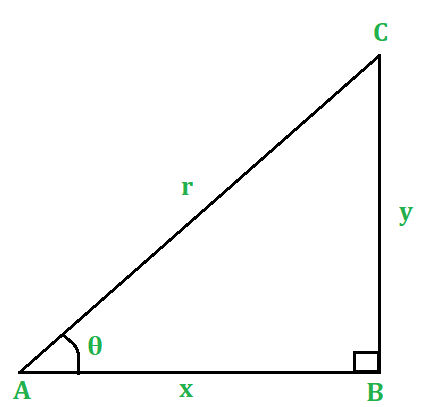# Trigonometric Identities

• Last Updated : 11 Feb, 2021

Trigonometry is that branch of Mathematics, which relates to the study of angles, measurement of angles, and units of measurement. It also concerns itself with the six ratios for a given angle and the relations satisfied by these ratios. In an extended way, the study is also of the angles forming the elements of a triangle. Logically, a discussion of the properties of a triangle; solving a triangle, physical problems in the area of heights and distances using the properties of a triangle – all constitute a part of the study. It also provides a method of solution of trigonometric equations.

### Trigonometric Identity

An equation involving trigonometric ratios of an angle is called trigonometric Identity if it is true for all values of the angle. These are useful whenever trigonometric functions are involved in an expression or an equation. The six basic trigonometric ratios are sine, cosine, tangent, cosecant, secant, and cotangent. All these trigonometric ratios are defined using the sides of the right triangle, such as an adjacent side, opposite side, and hypotenuse side.

Attention reader! All those who say programming isn't for kids, just haven't met the right mentors yet. Join the  Demo Class for First Step to Coding Coursespecifically designed for students of class 8 to 12.

The students will get to learn more about the world of programming in these free classes which will definitely help them in making a wise career choice in the future.

### Proof of the Trigonometric Identities

For any acute angle θ, prove that

(i) tanθ = sinθ/cosθ         (ii) cotθ = cosθ/sinθ            (iii) tanθ . cotθ = 1

(iv) sin2θ + cos2θ = 1       (v) 1 + tan2θ = sec2θ         (vi) 1 + cot2θ = cosec2θProof:

Consider a right-angled △ABC (fig. 1) in which ∠B = 90° and ∠A = 0°.

Let AB = x units, BC y units and AC = r units.

Then,

(i) tanθ = y/x = (y/r)/(x/r)     [dividing num. and denom. by r]

∴ tanθ = sinθ/cosθ

(ii) cotθ = x/y = (x/r)/(y/r)     [dividing num. and denom. by r]

∴ cotθ = cosθ/sinθ

(iii) tanθ . cotθ  = (sinθ/cosθ) . (cosθ/sinθ)

tanθ . cotθ = 1

Then, by Pythagoras’ theorem, we have

x2 + y2 = r2

Now,

(iv) sin2θ + cos2θ  = (y/r)2 + (x/r)2 = ( y2/r2 + x2/r2)

= (x2 + y2)/r2 = r2/r2 = 1 [x2+ y2 = r2]

sin2θ + cos2θ = 1

(v) 1 + tan2θ = 1 + (y/x)2 = 1 + y2/x2 = (y2 + x2)/x2 = r2/x2 [x2 + y2 = r2]

(r/x)2 = sec2θ

∴ 1 + tan2θ = sec2θ.

(vi) 1 + cot2θ = 1 + (x/y)2 = 1 + x2/y2 = (x2 + y2)/y2 = r2/y2 [x2 + y2 = r2]

(r2/y2) = cosec2θ

∴ 1 + cot2θ = cosec2θ.

### Application of Trigonometric Identites

Application 1: Prove that (1 – sin2θ) sec2θ = 1

Proof:

We have:

LHS = (1 – sin2θ) sec2θ

= cos2θ . sec2θ

= cos2θ . (1/cos2θ)

=1

= RHS.

∴ LHS = RHS.

Application 2: Prove that (1 + tan2θ) cos2θ = 1

Proof:

We have:

LHS = (1 + tan2θ)cos2θ

= sec2θ . cos2θ

= (1/cos2θ) . cos2θ

= 1 = RHS.

∴ LHS=RHS.

Application 3: Prove that (cosec2θ – 1) tan²θ  = 1

Proof:

We have:

LHS = (cosec²θ – 1) tan2θ

= (1 + cot2θ – 1) tan2θ

= cot2θ . tan2θ

= (1/tan2θ) . tan2θ

= 1 = RHS.

∴ LHS=RHS.

Application 4: Prove that (sec4θ – sec2θ) = (tan2θ + tan4θ)

Proof:

We have:

LHS = (sec4θ – sec2θ)

= sec2θ(sec2θ – 1)

= (1 + tan2θ) (1 + tan2θ – 1)

= (1 + tan2θ) tan2θ

= (tan2θ + tan4θ)

= RHS

∴ LHS = RHS.

Application 5: Prove that √(sec2θ + cosec2θ) = (tanθ + cotθ)

Proof:

We have:

LHS = √(sec2θ + cosec2θ ) = √((1 + tan2θ) + (1 + cot2θ))

= √(tan2θ + cot2θ + 2)

= √(tan2θ + cot2θ + 2tanθ.cotθ )         (tanθ . cotθ = 1)

= √(tanθ + cotθ)2

= tanθ + cotθ = RHS

∴ LHS = RHS.

My Personal Notes arrow_drop_up